## Algebra

Showing posts with label distributing. Show all posts
Showing posts with label distributing. Show all posts

### Simplifying Algebraic Expressions

The properties of real numbers are very important in our study of Algebra. These properties can be applied in Algebra because a variable is simply a letter that represents a real number. The distributive property is one that we apply often when simplifying algebraic expressions. Given real numbers a, b, c:
a(b + c) = ab + ac
When multiplying an expression within parentheses you must multiply everything inside by the number or variable that you are distributing.Introduction to the Distributive Property

Simplify.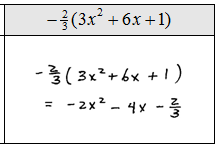When simplifying, we will often have to combine like terms after we distribute.  This step is consistent with the order of operations, multiplication before addition.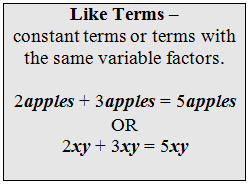Combining Like Terms

Simplify.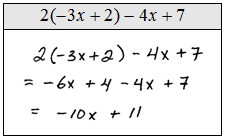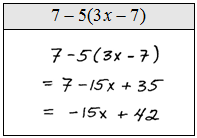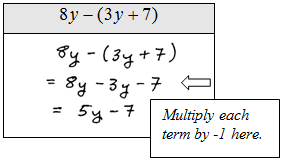Simplifying Algebraic Expressions

To combine like terms, the variable parts have to be exactly the same.  But before combining like terms, generally, we will first distribute if necessary.  When distributing negative numbers notice that the operations change.

Simplify.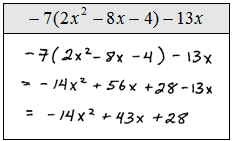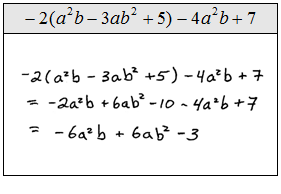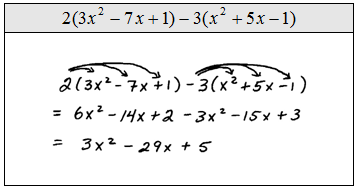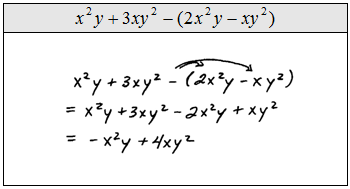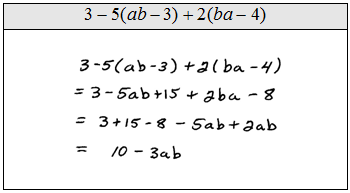Profit Word Problem: Profit is equal to revenues less cost of production.  If the revenue R can be represented byand the cost C can be represented bywhere x represents the number of units produced, find an equation that represents the profit.Subtracting Variable Expressions: What is the difference between 3x − 4 and −2x + 5?